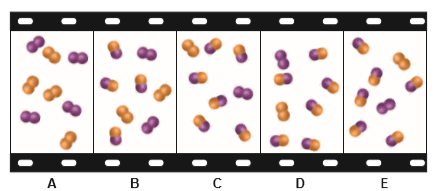# Problem: The “filmstrip” represents five molecular scenes of a gaseous mixture as it reaches equilibrium over time:X is purple and Y is orange: X2(g) + Y2(g) ⇌ 2XY(g). (a) Write the reaction quotient, Q, for this reaction.(b) If each particle represents 0.1 mol, find Q for each scene.

###### FREE Expert Solution

The reaction quotient, Q, can be calculated like an equilibrium constant with the formula shown below:

$\overline{){\mathbf{Q}}{\mathbf{=}}\frac{\mathbf{products}}{\mathbf{reactants}}}$

89% (440 ratings)###### Problem Details

The “filmstrip” represents five molecular scenes of a gaseous mixture as it reaches equilibrium over time:X is purple and Y is orange: X2(g) + Y2(g) ⇌ 2XY(g).

(a) Write the reaction quotient, Q, for this reaction.
(b) If each particle represents 0.1 mol, find Q for each scene.